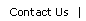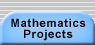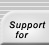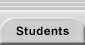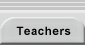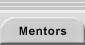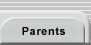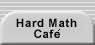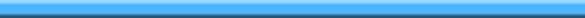Home > Mathematics Projects > Probabilistic Number Theory > Student Work > Noss (January 10, 2001)

Report on Work Done

Joe Noss
January 10, 2001

What is the probability that a randomly chosen integer has no square factors?

We can construct an initial formula to give us this value as follows:

If a number is to have no square factors, then it must not be a multiple of the square of any prime. The probability of a randomly chosen integer being a multiple of the square of a prime, p2 is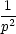since only 1 in every p2 numbers will belong to this times table.

Therefore, the probability of a number not being a multiple of this square prime is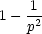So, taking the prime 5, the probability of a number not having 52 as a factor is 1 -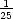=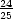.

In applying this to all integers, we must find the probability, P, that a number is not a multiple of the first prime squared, or the second prime squared, or the third prime squared. . . and so on.

This approach takes account of numbers which have the square of a composite number, such as four, as a factor. Taking 32 as an example, a multiple of 4 squared, we can see that according to the fundamental theorem of arithmetic, 4, a composite, can be given as the product of primes, in this case 2 × 2, and so 32 is also a multiple of the square of a prime, as is every number according to the theorem, so the method holds.

Given that we are asking the probability that a number bears a number of attributes (i.e. not being a multiple of any square prime) we must multiply the probability that each individual attribute applies together, to find the total probability.

Therefore,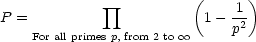Now, this product has an infinite number of terms.

Running a Logo model of the product reveals that it converges on around 0.603.

However, representing the product differently, makes it easier to evaluate also:

Think of evaluating the product: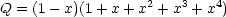Expanding out the brackets: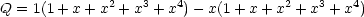From visualizing how these brackets will expand, it is obvious that everything will be cancelled except: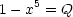Now in fact: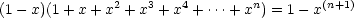Dividing by (1 - x):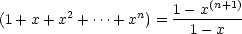x can of course be replaced by any number. So suppose it was replaced by r where -1 < r < 1. Then, as in differentiation from first principles, in the limit, rn+1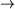0

Therefore, as n tends to infinity:So ,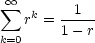when -1 < r < 1 Now let r =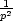(this is between -1 and 1)

Substituting this into the above: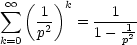So it seems our original component of that product, 1 -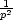, is now the denominator of this geometric series.

So now we know that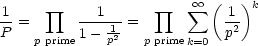So let’s look at that last way of representing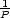. It would be written out as follows: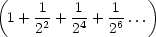×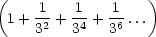×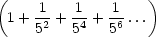×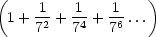×Of course, this is just the tip of the iceberg: every factor has an infinite number of terms, and there are an infinite number of factors. But suppose this was the entire product. To multiply it out, we would pick a term from each bracket, and multiply them together. We would continue with this, until we had covered every combination.

One combination would be: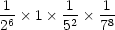Look at the denominators as they are multiplied together. The final product of the denominators will be the product of a number of squared primes.

Now the fundamental theorem of arithmetic states that every integer can be represented as the product of primes, e.g.:

Any integer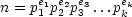Where the pi are primes and the ei are positive integers.

Now if we consider n2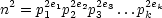as we have in our denominators.

So according to the fundamental theorem of arithmetic, we should be able to obtain any square number as the denominator of one of the products.

Furthermore, the theorem states that each number can only be represented uniquely by primes, and so this suggests that every number can only appear once. Therefore,can again be restated as the series:1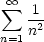Where n is every integer from one. . .

Now a quick Logo program reveals this converges onto around 1.64046 which is the reciprocal of the original value.

1 This is not a geometric sequence --this can be seen by the ratio between the terms not being constant.Translations of mathematical formulas for web display were created by tex4ht. © Copyright 2003 Education Development Center, Inc. (EDC)